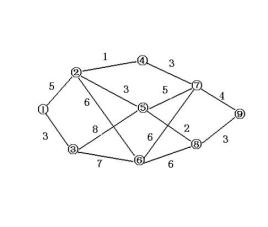# Optimal path algorithm

## Basic Principles

A path is a path in which an undirected graph satisfies all the vertices (except the start point and the end point) on the path are different, and all the edges are also different. The optimal path is the path that best meets a certain requirement among the paths. For example, the shortest path is the path with the smallest edge weight from the start point to the end point. The method of finding the optimal path for the graph is called the optimal path algorithm, which is usually implemented by computer programming.

## Application

In road transportation, in order to minimize the time and cost of transportation (the cost can be fuel consumption and expense), it is necessary to find the optimal path for the starting point and the ending point. This optimal path can be the shortest path or the path with the least fuel consumption, depending on actual demand. In practical applications, knowing the starting point and ending point, the optimal path algorithm can be used to calculate the path, which has a good guiding effect on the driving of the vehicle. The use of computer programming can improve efficiency. However, similar systems are rare. Among them, ptystudio (road transportation cost calculator) can calculate the transportation cost between any two places in China. In use, just enter the starting point, destination, vehicle type and other information, and ptystudio can calculate the current refined oil price and highway Tolls on highways calculate transportation costs, including details such as fuel consumption, mileage, and highway tolls. The calculation of ptystudio is based on the system of calculating the optimal path. The calculated cost is based on the shortest path between the two places. However, the calculation of the path uses the optimal path algorithm, which uses computers to realize automatic calculation.Related Articles